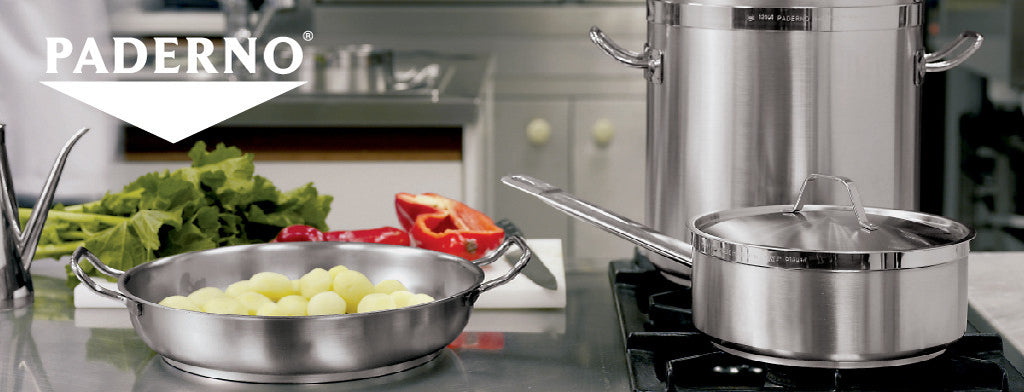Shopping Cart

### Refine

View all

#### Price£30.90 each (ex VAT)£27.04 each (ex VAT)£21.87 each (ex VAT)£46.35 each (ex VAT)£87.57 each (ex VAT)£124.81 each (ex VAT)£37.35 each (ex VAT)£33.19 each (ex VAT)£54.09 each (ex VAT)£27.06 each (ex VAT)£19.33 each (ex VAT)£28.42 each (ex VAT)£22.73 each (ex VAT)£18.66 each (ex VAT)£12.88 each (ex VAT)£11.60 each (ex VAT)£20.60 each (ex VAT)£16.75 each (ex VAT)£464.00 each (ex VAT)£707.00 each (ex VAT)£807.00 each (ex VAT)£1,100.00 each (ex VAT)£59.89 each (ex VAT)£23.17 each (ex VAT)£30.90 each (ex VAT)£37.33 each (ex VAT)£12.86 each (ex VAT)£16.73 each (ex VAT)£25.11 each (ex VAT)£45.06 each (ex VAT)£37.31 each (ex VAT)£38.62 each (ex VAT)£18.67 each (ex VAT)£23.17 each (ex VAT)£31.55 each (ex VAT)£13.51 each (ex VAT)£12.86 each (ex VAT)£20.59 each (ex VAT)£24.56 each (ex VAT)£24.45 each (ex VAT)£29.60 each (ex VAT)£38.62 each (ex VAT)